JEE  >  NCERT Solutions Exercise 5.5: Continuity & Differentiability

# NCERT Solutions Exercise 5.5: Continuity & Differentiability - Mathematics (Maths) for JEE Main & Advanced

Continuity & Differentiability

Question 1: Differentiate the function with respect to x.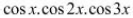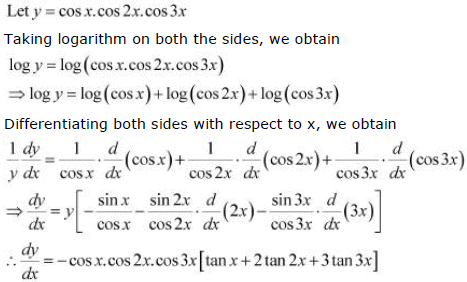Question 2: Differentiate the function with respect to x.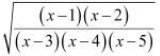Let y =Taking logarithm on both the sides, we obtain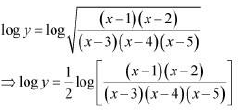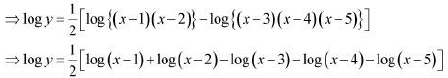Differentiating both sides with respect to x, we obtain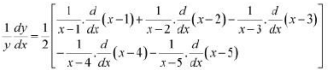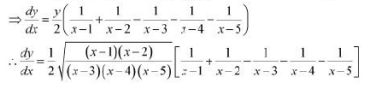Question 3: Differentiate the function with respect to x.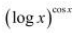Let y =Taking logarithm on both the sides, we obtain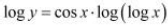Differentiating both sides with respect to x, we obtain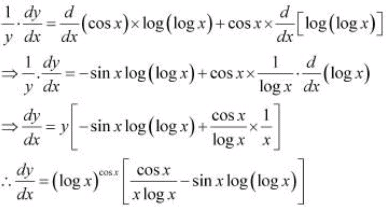Question 4: Differentiate the function with respect to x. xx - 2sinx

Let y =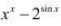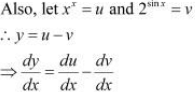u = xx
Taking logarithm on both the sides, we obtain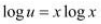Differentiating both sides with respect to x, we obtain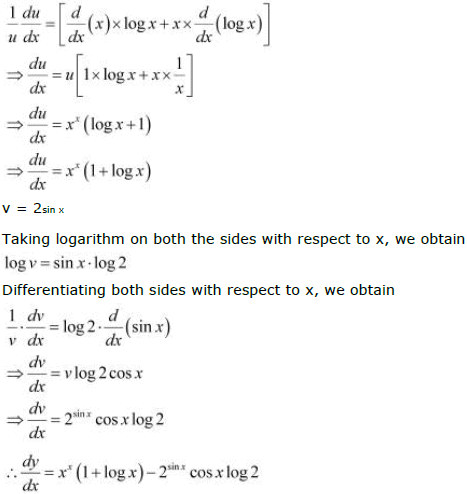Question 5: Differentiate the function with respect to x.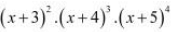Let y =Taking logarithm on both the sides, we obtain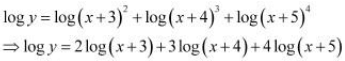Differentiating both sides with respect to x, we obtain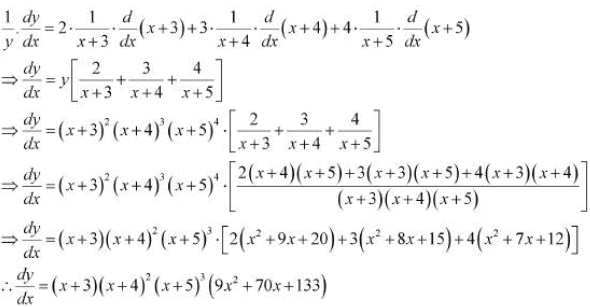Question 6: Differentiate the function with respect to x.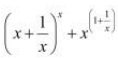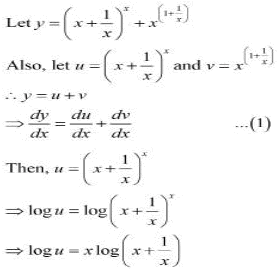Differentiating both sides with respect to x, we obtain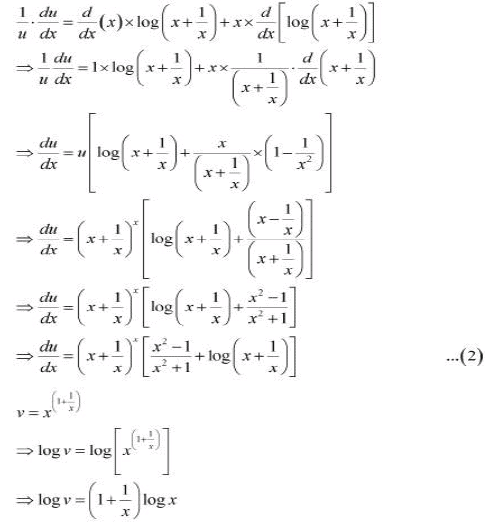Differentiating both sides with respect to x, we obtain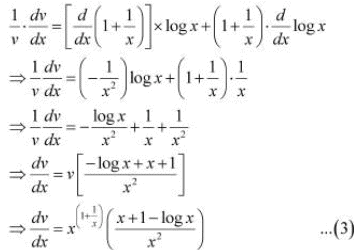Therefore, from (1), (2), and (3), we obtain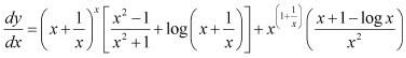Question 7: Differentiate the function with respect to x.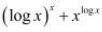Let y =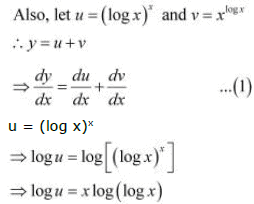Differentiating both sides with respect to x, we obtain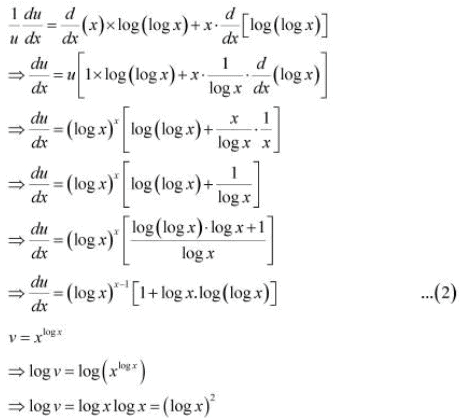Differentiating both sides with respect to x, we obtain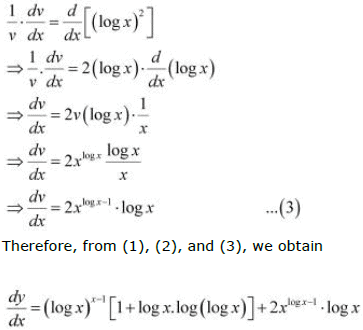Question 8: Differentiate the function with respect to x.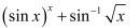Let y =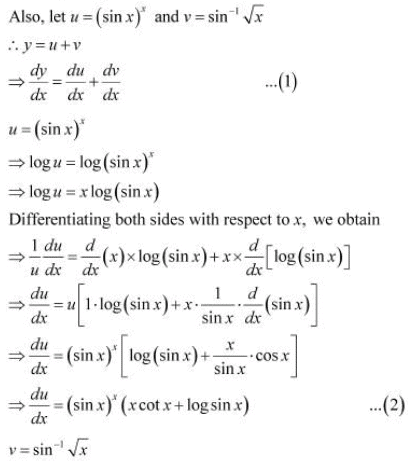Differentiating both sides with respect to x, we obtain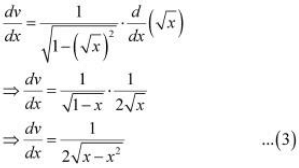Therefore, from (1), (2), and (3), we obtain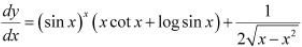Question 9: Differentiate the function with respect to x.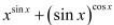Let y =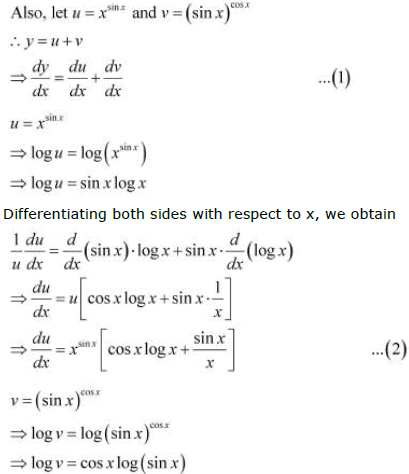Differentiating both sides with respect to x, we obtain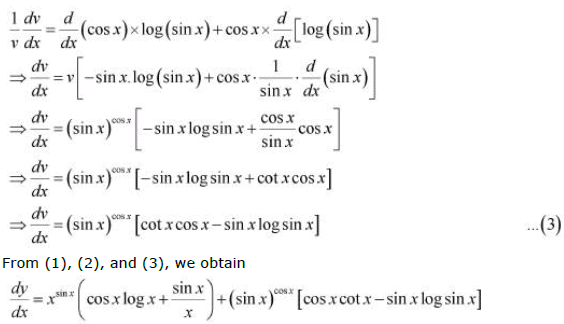Question 10: Differentiate the function with respect to x.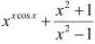Let y =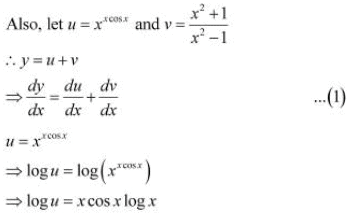Differentiating both sides with respect to x, we obtain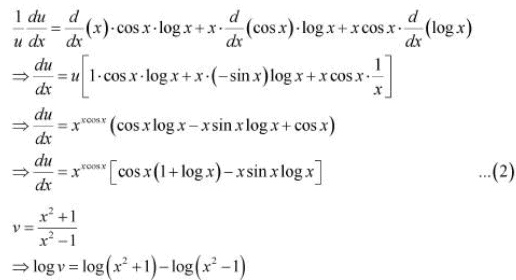Differentiating both sides with respect to x, we obtain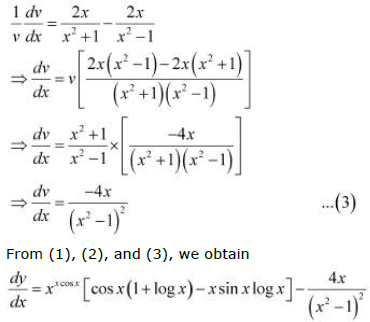Question 11: Differentiate the function with respect to x.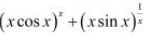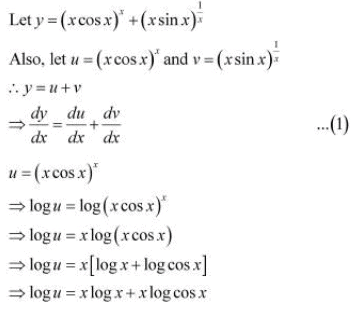Differentiating both sides with respect to x, we obtain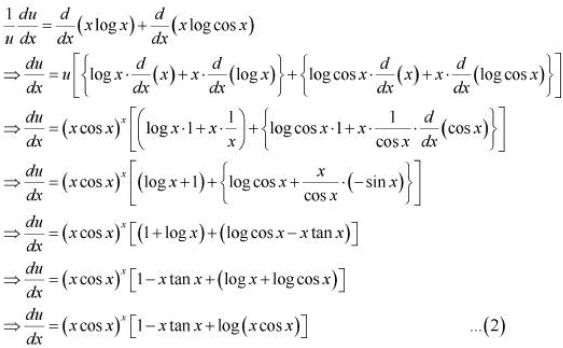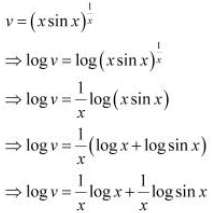Differentiating both sides with respect to x, we obtain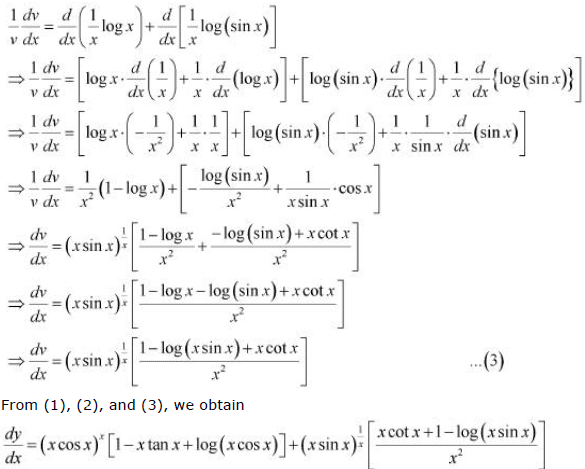Question 12:
Find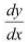of function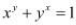.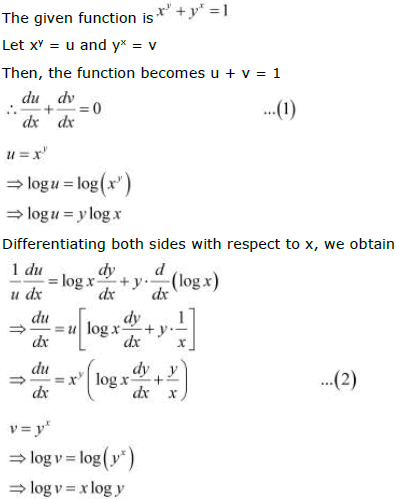Differentiating both sides with respect to x, we obtain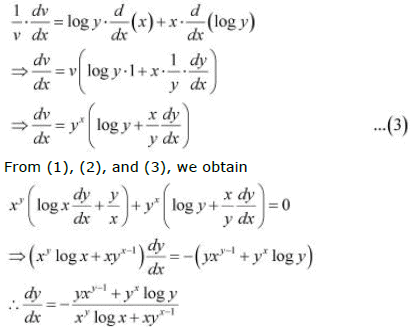Question 13:

Find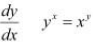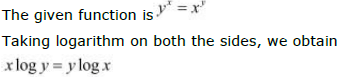Differentiating both sides with respect to x, we obtain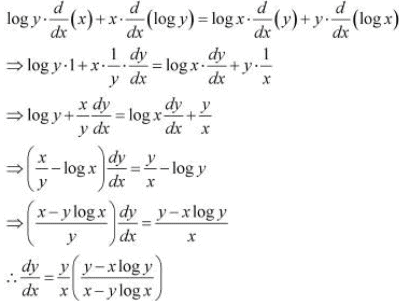Question 14:
Findof function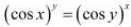.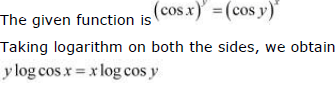Differentiating both sides, we obtain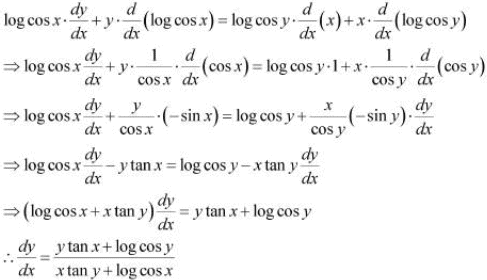Question 15:
Findof function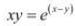.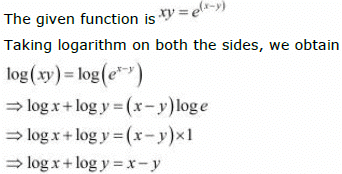Differentiating both sides with respect to x, we obtain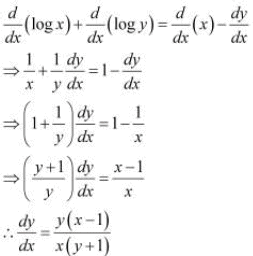Question 16:
Find the derivative of the function given by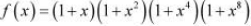and
hence find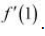.

The given relationship isTaking logarithm on both the sides, we obtain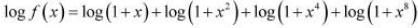Differentiating both sides with respect to x, we obtain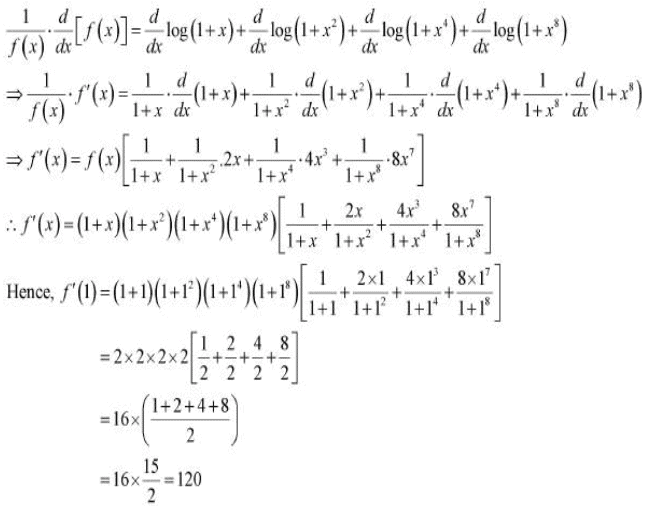Question 17:
Differentiate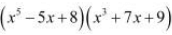in three ways mentioned below
(i) By using product rule.
(ii) By expanding the product to obtain a single polynomial.
(iii By logarithmic differentiation.
Do they all give the same answer?

Let y =(i)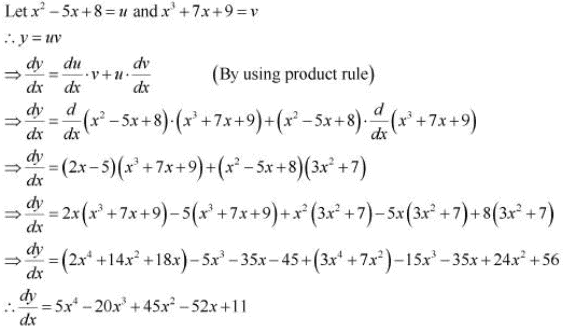(ii)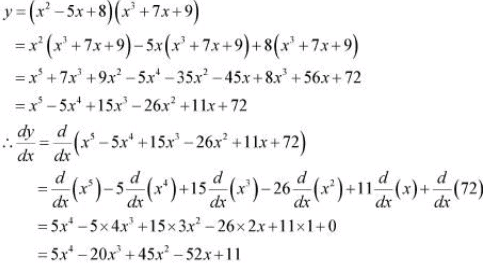( iii)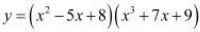Taking logarithm on both the sides, we obtain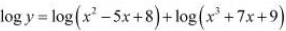Differentiating both sides with respect to x, we obtain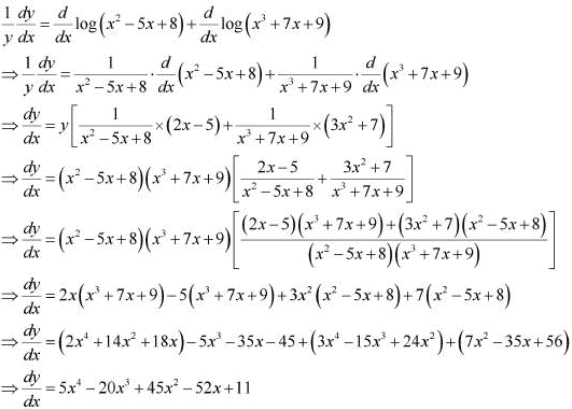From the above three observations, it can be concluded that all the results ofare same.

Question 18: If u, v and w are functions of x, then show that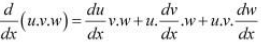in two ways-first by repeated application of product rule, second by logarithmic
differentiation.

Let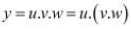By applying product rule, we obtain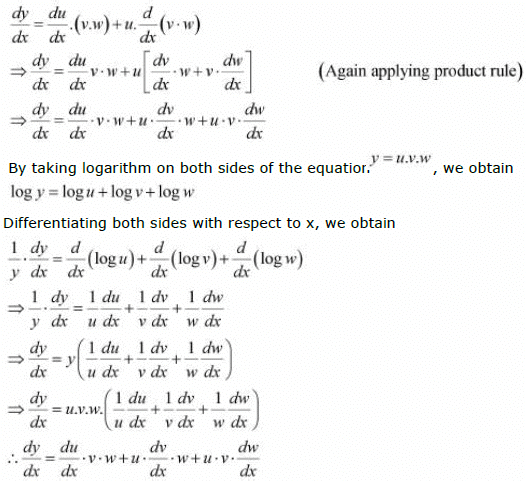The document NCERT Solutions Exercise 5.5: Continuity & Differentiability | Mathematics (Maths) for JEE Main & Advanced is a part of the JEE Course Mathematics (Maths) for JEE Main & Advanced.
All you need of JEE at this link: JEE

## FAQs on NCERT Solutions Exercise 5.5: Continuity & Differentiability - Mathematics (Maths) for JEE Main & Advanced

 1. What is continuity in calculus?Ans. Continuity refers to the property of a function where there are no abrupt jumps, breaks, or holes in the graph. It means that the function is smooth and can be drawn without lifting the pen from the paper. In simpler terms, a function is continuous if its graph can be traced without any interruptions.
 2. What does differentiability mean in calculus?Ans. Differentiability is a property of a function where it has a derivative at every point in its domain. It implies that the function is smooth and its graph doesn't have sharp turns or corners. A differentiable function can be approximated well by a straight line at any point on its graph.
 3. How are continuity and differentiability related?Ans. Continuity is a necessary condition for differentiability. If a function is differentiable at a point, then it must also be continuous at that point. However, continuity alone doesn't guarantee differentiability. A function can be continuous but not differentiable if there is a sharp corner or vertical tangent at a particular point.
 4. How can we determine if a function is continuous?Ans. To determine if a function is continuous, we need to check three conditions: 1. The function should be defined at the given point. 2. The limit of the function as it approaches the given point should exist. 3. The value of the function at the given point should be equal to the limit. If all three conditions are satisfied, then the function is continuous at that point.
 5. How do we test for differentiability of a function?Ans. To test for differentiability of a function, we need to check if the derivative exists at each point in its domain. The derivative can be calculated using differentiation rules or formulas. If the derivative exists for all points in the domain, then the function is differentiable. However, if there are any points where the derivative doesn't exist, then the function is not differentiable at those points.

## Mathematics (Maths) for JEE Main & Advanced

129 videos|359 docs|306 tests

## Mathematics (Maths) for JEE Main & Advanced

129 videos|359 docs|306 tests
Signup to see your scores go up within 7 days! Learn & Practice with 1000+ FREE Notes, Videos & Tests.
10M+ students study on EduRev
Track your progress, build streaks, highlight & save important lessons and more!(Scan QR code)
Related Searches

,

,

,

,

,

,

,

,

,

,

,

,

,

,

,

,

,

,

,

,

,

;